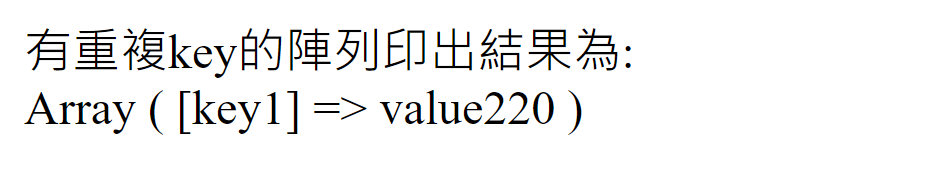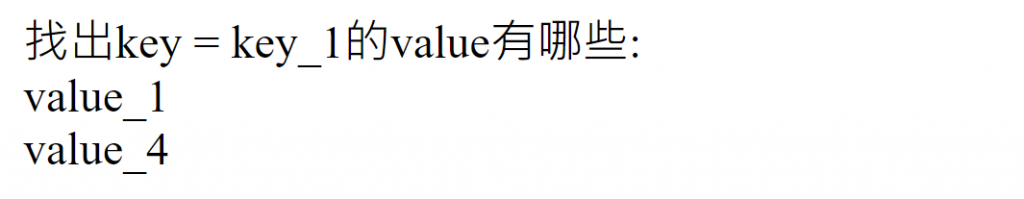#DAY 13
0

## Day 13 : PHP - 當陣列中有兩個重複的key值，該如何將它們的value全部印出？

PHP:

``````//宣告一個一維陣列，其key1重複兩次
\$one_array = array("key1" => "value110", "key1" => "value220");
echo "有重複key的陣列印出結果為:<br>";
print_r(\$one_array);
``````PHP:
(注意：這裡有兩個重複的key`key_1`)

``````//宣告一個二維陣列，其key_1重複兩次
\$many_array = array(array("key_1" => "value_1"), //key = 0
array("key_2" => "value_2"), //key = 1
array("key_3" => "value_3"), //key = 2
array("key_1" => "value_4")); //key = 4
``````

PHP:

``````//欲印出value的key值
\$searchKey = "key_1";

echo "找出key = key_1的value有哪些:<br>";

//這裡只需定義value，因為我們沒定義key值，所以key值會自動從0開始
//mValue = \$many_array裡的陣列
foreach (\$many_array as \$mValue) {
foreach (\$mValue as \$key => \$value) {
//找出和\$searchKey相同的\$key值
if (\$key == \$searchKey) {
echo \$value.'<br>';
}
}
}
``````